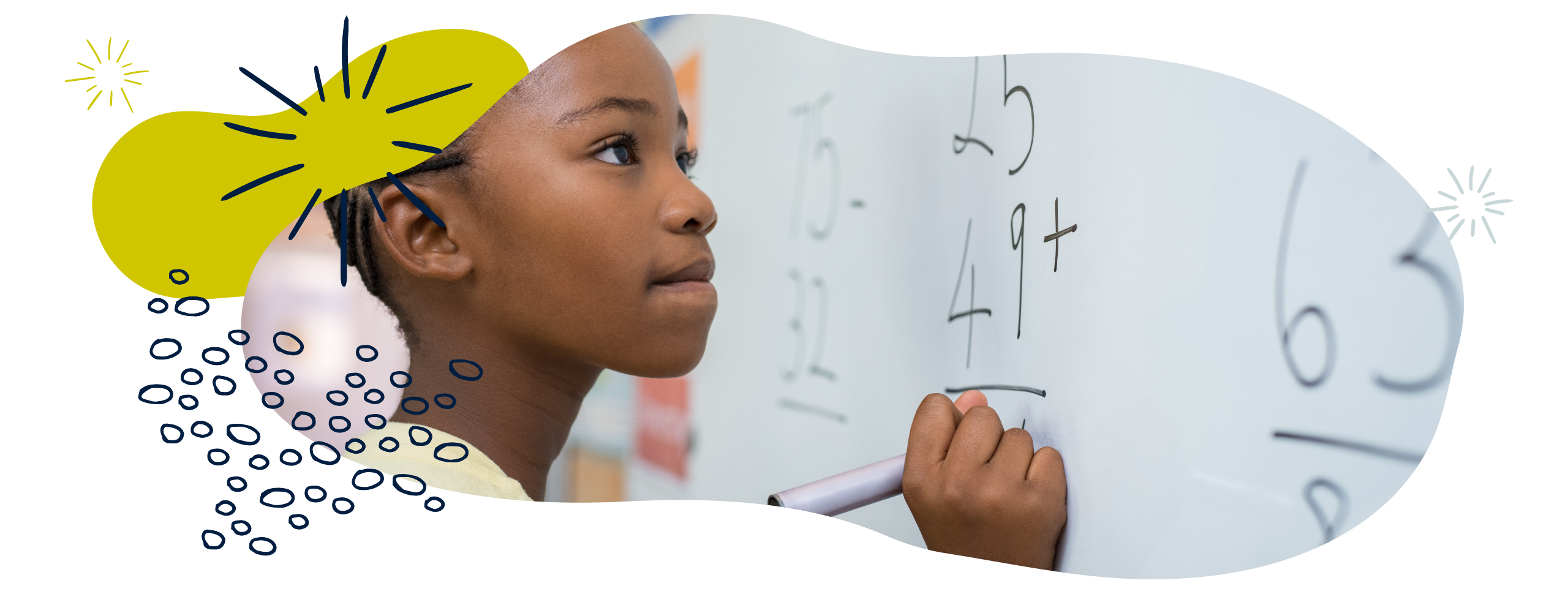We use cookies to enhance your experience on our website. By continuing to use our website, you are agreeing to our use of cookies. You can change your cookie settings at any time. Find out moreWe all feel confident talking about addition and subtraction. From counting on our fingers to moving along a number line, learning to add and subtract is one of the first maths skills that children will learn in school.

Although this may sound simple, understanding the relationship between numbers and what happens when you add and subtract numbers is quite a complex skill.

## Maths glossary

Use these quick links or explore our education glossary for definitions and examples of mathematical terms.

• The area/grid method method is a way of visualising multiplication, as part of formal calculations. It involves breaking numbers up and multiplying their parts separately in a grid, before adding them back together.
• Inverse operations are operations that can ‘undo’ each other. Addition is the inverse of subtraction.
• Number bonds are pairs that make up a total. For example, the number bonds for 4 are 0 + 4, 1 + 3, and 2 + 2.
• Number lines are images used to help children grasp number relationships. You can use them to count on or back to solve addition or subtraction problems.
• Partitioning means to split a number into smaller chunks. It is often used to break down larger numbers to make calculations easier.

Search our education glossary

You don’t need to be an expert to support your child with maths! To help you on your way, maths expert Kate Robinson shares some simple tips to show you how you can support your young mathematician at home:

Maths expert Kate Robinson explains how children develop their addition skills using real objects like counters and toys.

### Early maths skills: subtraction

Maths expert Kate Robinson explains how your child can learn to subtract by solving everyday maths problems.

## What your child will learn at school

#### Addition & subtraction in Year 1 (age 5–6)

In Year 1, your child will be expected to be able to read, write, and understand mathematical ideas using addition (+), subtraction (–) and equals (=) signs. This includes:

• making and using number bonds to 10 and then to 20
• adding and subtracting one-digit and two-digit numbers to 20, including 0
• solving simple problems using objects, drawings, diagrams and symbols, including missing number problems such as 7 = ? – 9.

#### Addition & subtraction in Year 2 (age 6–7)

In Year 2, your child will be expected to be able to solve addition and subtraction problems using numbers with one and two digits. This includes:

• knowing and using addition and subtraction facts up to 20 and working out related addition and subtraction facts up to 100
• adding and subtracting using objects, pictures, and drawings, and also solving problems mentally
• understanding that addition and subtraction have an inverse relationship (i.e. they undo each other), and using this to check calculations.

#### Addition & subtraction in Year 3 (age 7–8)

In Year 3, your child will be expected to use a range of strategies to solve problems mentally, and to learn formal written methods for column addition and column subtraction. This includes:

• adding and subtracting numbers with up to three digits
• estimating answers to problems before working them out accurately and checking using the inverse operation (i.e. using addition to check subtraction and vice versa)
• explaining how they have solved a problem and why they chose a particular method.

#### Addition & subtraction in Year 4 (age 8–9)

In Year 4, your child will be expected to be able to solve addition and subtraction problems involving numbers up to four digits. This includes:

• choosing from a variety of methods, including mental calculations, using objects, diagrams and drawings such as number lines, the area/grid method, and written column addition and subtraction
• estimating answers before calculating accurately and checking answers by understanding that addition and subtraction are inverse operations
• solving two-step word problems that require them to solve two different calculations to get the answer.

#### Addition & subtraction in Year 5 (age 9–10)

In Year 5, your child will be expected to be able to solve addition and subtraction problems involving numbers with more than four digits. This includes:

• practising a range of mental calculation strategies and a variety of formal calculation methods, like using objects, diagrams and drawings such as number lines, the area/grid method, and written column addition and subtraction
• using rounding to estimate answers and checking that their answers are sensible and accurate
• solving multi-step word problems that involve multiple calculations before coming to the final answer.